21.1
20.2
20.1
19.2
19.1
18.2
18.1
17.2
A newer version of this page is available. Switch to the current version.

# PivotGridFieldBase.SummaryType Property

Gets or sets the type of the summary function which is calculated against the current data field.

Namespace: DevExpress.XtraPivotGrid

Assembly: DevExpress.PivotGrid.v18.2.Core.dll

## Declaration

``````[DefaultValue(PivotSummaryType.Sum)]
[XtraSerializableProperty]
[XtraSerializablePropertyId(2)]
public PivotSummaryType SummaryType { get; set; }``````

## Property Value

Type Default Description
PivotSummaryType

Sum

A PivotSummaryType enumeration value that specifies the summary function type which is calculated against the current field.

Available values:

Name Description
Count

The number of values (excluding Null and DBNull values).

Sum

The sum of the values.Min

The smallest value.

Max

The largest value.

Average

The average of the values.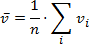StdDev

An estimate of the standard deviation of a population, where the sample is a subset of the entire population.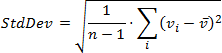StdDevp

The standard deviation of a population, where the population is all of the data to be summarized.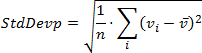Var

An estimate of the variance of a population, where the sample is a subset of the entire population.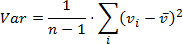Varp

The variance of a population, where the population is all of the data to be summarized.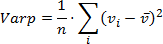Custom

Specifies whether calculations should be performed manually, by handling the CustomSummary event.

Not supported in OLAP and server mode.

## Remarks

PivotGridControl calculates summaries against data fields. The summary function type is determined by the field’s SummaryType property.

For fields which don’t contain numeric data only the following summary functions can be calculated: PivotSummaryType.Count, PivotSummaryType.Min and PivotSummaryType.Max. If the field’s SummaryType property is set to any other value the corresponding data cells will display zeros.

For numeric data fields any summary function type listed by the PivotSummaryType enumerator can be calculated.

##### NOTE

The SummaryType property is not in effect in the OLAP mode. The summary type of a measure is controlled by Analysis Services itself and it is not possible to customize it using the PivotGrid control.

See Also